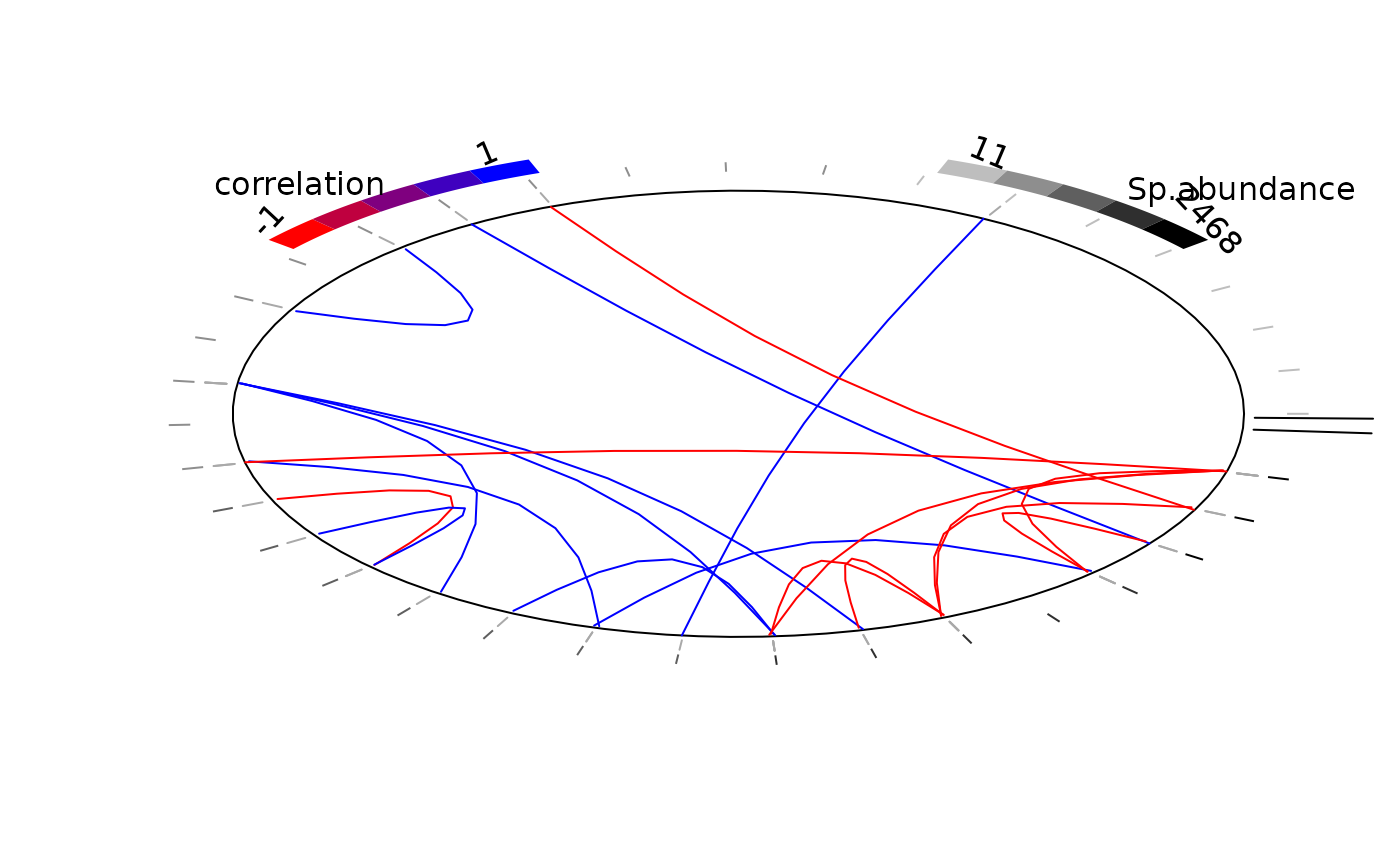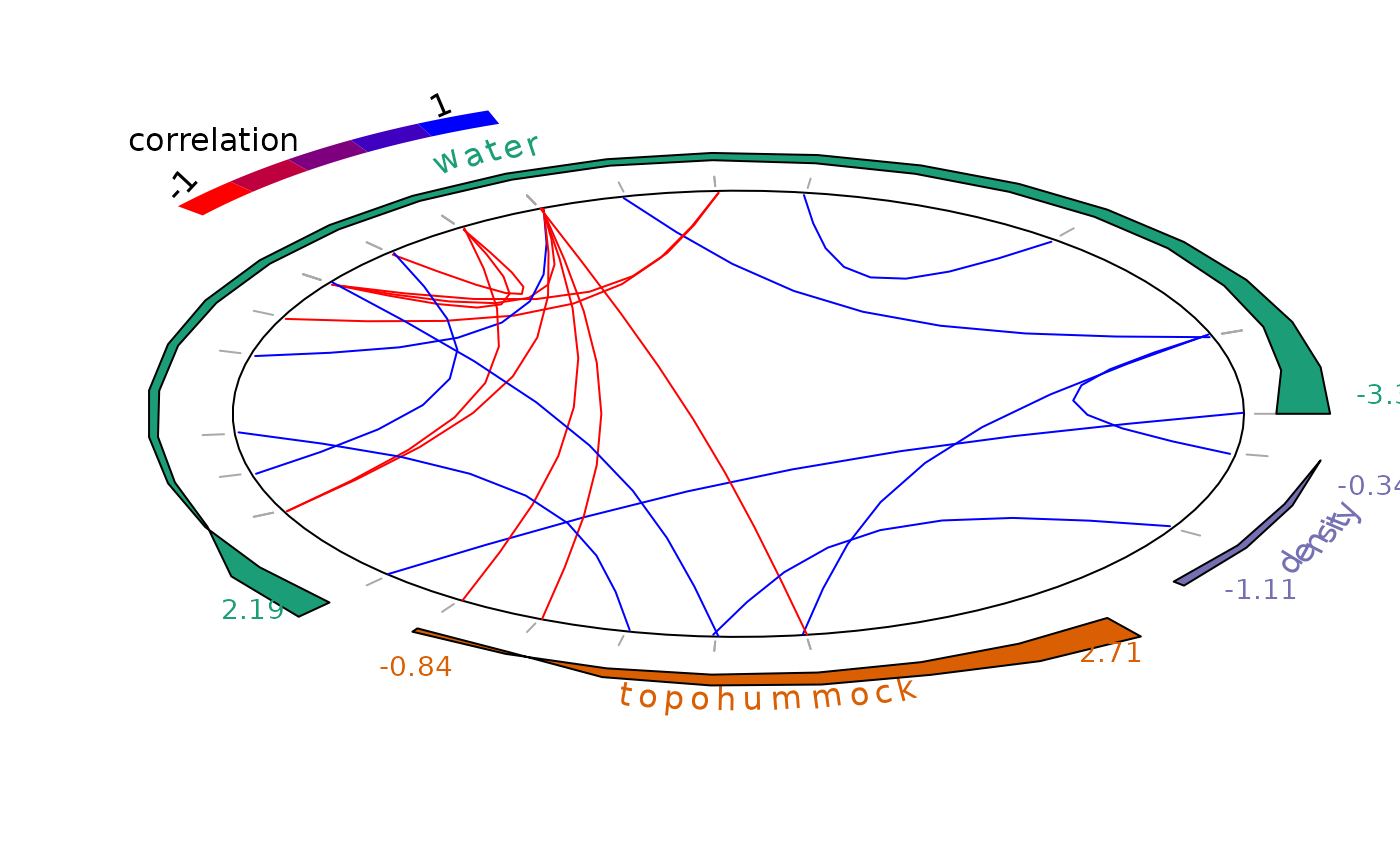plot_associations plot species-species associations

plot_associations(
R,
main = NULL,
cex.main = NULL,
circleBreak = FALSE,
top = 10L,
occ = NULL,
env_effect = NULL,
cols_association = c("#FF0000", "#BF003F", "#7F007F", "#3F00BF", "#0000FF"),
cols_occurrence = c("#BEBEBE", "#8E8E8E", "#5F5F5F", "#2F2F2F", "#000000"),
cols_env_effect = c("#1B9E77", "#D95F02", "#7570B3", "#E7298A", "#66A61E", "#E6AB02",
"#A6761D", "#666666"),
lwd_occurrence = 1,
species_order = "abundance",
species_indices = NULL
)

## Arguments

R

matrix of correlation $$R$$

main

title

cex.main

title's character size. NULL and NA are equivalent to 1.0.

circleBreak

circle break or not

top

number of top negative and positive associations to consider

occ

species occurence data

env_effect

environmental species effects $$\beta$$

cols_association

cols_occurrence

cols_env_effect

lwd_occurrence

lwd for occurrence lines

species_order

order species according to :

 "abundance" their mean abundance at sites by default) "frequency" the number of sites where they occur "main environmental effect" their most important environmental coefficients
species_indices

indices for sorting species

## Details

After fitting the jSDM with latent variables, the fullspecies residual correlation matrix : $$R=(R_{ij})$$ with $$i=1,\ldots, n_{species}$$ and $$j=1,\ldots, n_{species}$$ can be derived from the covariance in the latent variables such as : can be derived from the covariance in the latent variables such as : $$\Sigma_{ij}=\lambda_i' .\lambda_j$$, in the case of a regression with probit, logit or poisson link function and

 $$\Sigma_{ij}$$ $$= \lambda_i' .\lambda_j + V$$ if i=j $$= \lambda_i' .\lambda_j$$ else,

this function represents the correlations computed from covariances : $$R_{ij} = \frac{\Sigma_{ij}}{\sqrt{\Sigma_ii\Sigma _jj}}$$.

jSDM-package get_residual_cor
jSDM_binomial_probit jSDM_binomial_probit_long_format
jSDM_binomial_probit_sp_constrained jSDM_binomial_logit jSDM_poisson_log

## Author

Jeanne Clément <jeanne.clement16@laposte.net>

## Examples

library(jSDM)
# frogs data
data(mites, package="jSDM")
# Arranging data
PA_mites <- mites[,1:35]
# Normalized continuous variables
Env_mites <- cbind(mites[,36:38], scale(mites[,39:40]))
colnames(Env_mites) <- colnames(mites[,36:40])
Env_mites <- as.data.frame(Env_mites)
# Parameter inference
# Increase the number of iterations to reach MCMC convergence
mod <- jSDM_poisson_log(# Response variable
count_data=PA_mites,
# Explanatory variables
site_formula = ~  water + topo + density,
site_data = Env_mites,
n_latent=2,
site_effect="random",
# Chains
burnin=100,
mcmc=100,
thin=1,
# Starting values
alpha_start=0,
beta_start=0,
lambda_start=0,
W_start=0,
V_alpha=1,
# Priors
shape=0.5, rate=0.0005,
mu_beta=0, V_beta=10,
mu_lambda=0, V_lambda=10,
# Various
seed=1234, verbose=1)
#>
#> Running the Gibbs sampler. It may be long, please keep cool :)
#>
#> **********:10.0%, mean accept. rates= beta:0.206 lambda:0.191 W:0.331 alpha:0.216
#> **********:20.0%, mean accept. rates= beta:0.245 lambda:0.229 W:0.254 alpha:0.201
#> **********:30.0%, mean accept. rates= beta:0.326 lambda:0.286 W:0.282 alpha:0.261
#> **********:40.0%, mean accept. rates= beta:0.344 lambda:0.344 W:0.366 alpha:0.354
#> **********:50.0%, mean accept. rates= beta:0.400 lambda:0.397 W:0.378 alpha:0.389
#> **********:60.0%, mean accept. rates= beta:0.409 lambda:0.438 W:0.408 alpha:0.410
#> **********:70.0%, mean accept. rates= beta:0.404 lambda:0.428 W:0.397 alpha:0.394
#> **********:80.0%, mean accept. rates= beta:0.412 lambda:0.414 W:0.388 alpha:0.429
#> **********:90.0%, mean accept. rates= beta:0.435 lambda:0.425 W:0.386 alpha:0.433
#> **********:100.0%, mean accept. rates= beta:0.412 lambda:0.424 W:0.382 alpha:0.425
# Calcul of residual correlation between species
R <- get_residual_cor(mod)$cor.mean plot_associations(R, circleBreak = TRUE, occ = PA_mites, species_order="abundance")# Average of MCMC samples of species enrironmental effect beta except the intercept env_effect <- t(sapply(mod$mcmc.sp,
colMeans)[grep("beta_", colnames(mod\$mcmc.sp[]))[-1],])
colnames(env_effect) <-  gsub("beta_", "", colnames(env_effect))
plot_associations(R, env_effect = env_effect, species_order="main env_effect")